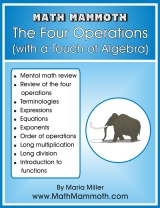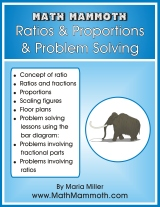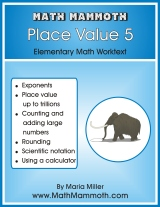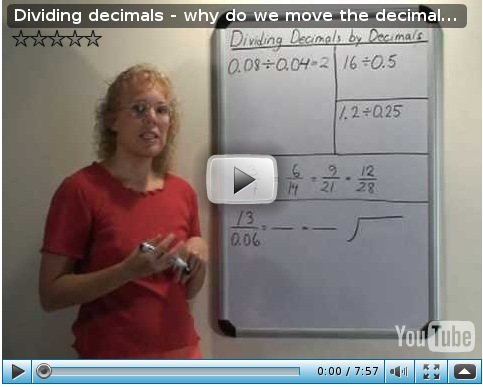# Homeschool Math Newsletter, Vol. 38, March 2010Math practice has never been so much fun! Practice math at IXL, the world's most complete math practice site for Pre-K through middle school. Excel in math... IXL.com

## 1. New Math Mammoth books

There are now three new books available in the Blue Series:

1.### Math Mammoth The Four Operations (with a Touch of Algebra)

The main topics studied in this book are simple equations, expressions that involve a variable, the order of operations, long multiplication, long division, and graphing simple linear functions.

The idea is not to practice each of the four operations separately, but rather to see how they are used together in solving problems and in simple equations. We are trying to develop student's algebraic thinking. Many of the ideas in this chapter are preparing them for algebra in advance.

2. ### Math Mammoth Ratios & Proportions & Problem SolvingThis worktext concentrates, first of all, on two important concepts: ratios and proportions, and then on problem solving.

First, we study thoroughly the concept of ratio, including how it connects with fractions and the aspect ratio in rectangles.

Solving proportions is divided into three separate lessons. In the first one, we solve proportions by thinking through equivalent ratios. In the second one, the usual method of cross-multiplying is introduced. Then follows a lesson that explains just why cross-multiplying is allowed. Then there is more practice with solving proportions and word problems. We also study scaling geometric figures and floor plans.

The last lessons concentrate on various kinds of word problems that can be solved with the help of bar or block diagrams (the same kind as in Singapore Math). These involve problems with fractional parts, and then problems involving ratios. The diagrams become a powerful tool to solve such problems without the use of algebra.

3.### Math Mammoth Place Value 5

Math Mammoth Place Value 5 is a short worktext that covers value up to the trillions—that is, numbers up to 15 digits&mdashrounding them, and using a calculator. The first lesson covers place value up to billions. Then, we study exponents, and right after that, place value up to trillions, writing the numbers in the expanded form using exponents. After working with addition a little, there are two lessons about calculator usage.

Light Blue grade 6-A is also around the corner - should be here by the end of March!

## 2. Divide decimals by decimals - why do we move the decimal point?

When dividing decimals by decimals, such as 45.89 ÷ 0.006, we are told to move the decimal point in both the dividend and the divisor so many steps that the divisor becomes a whole number. Then, you use long division. But why?

Schoolbooks often don't tell us the "why", just the "how".

This video explores this concept.It has to do with the fact that when we move the decimal point, we are multiplying both numbers by 10, 100, 1000, or some other power of ten. When the dividend and the divisor are multiplied by the same number, the quotient does not change. This principle makes sense:

0.344 ÷ 0.004 can be thought of, "How many times does four thousandths fit into 344 thousandths?"

The same number of times as what four fits into 344!

So, 0.344 ÷ 0.004 can be changed into the division problem 344 ÷ 4 without changing the answer. Both 0.344 and 0.004 got multiplied by 1000.

When we simplify fractions or write equivalent fractions, we use the same principle. Remember, fractions are like division problems.

3/7 is 3 ÷ 7.

And, 3/7 = 6/14. We can multiply the numerator and the denominator (or the dividend and the divisor) by some same number, without changing the value of the fraction (the quotient).

Or, 90/100 = 9/10. Or 0.9/0.2 = 9/2.

## 3. A plane in still air - algebra problem

Someone sent me this algebra problem:

An airplane flew for 6 hours with a tail wind of 60km/hr. The return flight against the same wind took 8 hours. Find the speed of the boat in still water.

Initially, this sounds like a trick problem, because you can't know the speed of the boat when all the information given is about an airplane!

But, let's assume they meant to ask the speed of the AIRPLANE in still air.

This problem has to do with constant speed. Constant speed ALWAYS involves TIME & DISTANCE.

And here we have two situations with two different speeds: First the airplane flies over there, with the wind helping. Then it flies back, and the wind is contrary of course.

It helps to organize our information in a table, once again. We'll need time, distance, and speed. And remember, speed = distance/time, or distance = speed * time. This time, time is known for both situations. Distance is not, but it is the same distance that way and back (call it d). Traveling there, the speed will be the sum of the airplane's speed (v) plus the wind speed (60). Traveling back, the wind is contrary so its speed is subtracted from the airplane's speed.

## 4. Tidbits

• Free exponent worksheets
Create an unlimited supply of free printable exponents worksheets there. The worksheets concentrate on calculations with exponents, such as solving 33 or (1/2)4 or (-5)0 or 8-2. You can choose to include negative or zero exponent. You can choose fractions, decimals, or negative numbers as bases. You can also make worksheets that have one other operation besides exponentiation (several operations with powers).

• World Math Day
World Math Day 2010 is coming up on March 3rd. You might remember it from last year. It is a free event and lots of fun for the kids. Registrations close March 2nd - TODAY! HURRY! Once you register, you can go practice at the website.

• Pi Day
Pi day is March 14 (3/14). To celebrate it and have some activities for your children/students, check these resources.

• Basics of the number e (was: celebrating e-day)
e or Euler's number is a number that is approximately 2.718281828, so that is why someone chose 2/7 as an e-day. But e is an irrational number, so its decimal expansion is never-ending and never-repeating. Why is this number e so important that people have even named a day after it?

Till next month,
Maria Miller

Miss something from the earlier volumes? See newsletter archives.

Feel free to forward this issue to a friend. Subscribe here.

 Home    Blog    Math Mammoth books    Math Worksheets    Curriculum Guide    Newsletter Archives Lessons & Teaching Tips    Reviews    Online Math Games & Tutorials